# Velocity - 7th grade (12y) - math problems

#### Number of problems found: 152

• Change per hour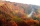On Sunday, the temperature was 63 degrees at 2 pm. The temperature was then 72 degrees at 5 pm. What is the rate of change per hour?
• The carThe car has a consumption of 5 liters per 100 km. It has 24 liters of gasoline in the tank. How much petrol remained in the tank when it rides for two and a half hours at a speed of 80 km/h?
• A giraffe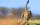A giraffe can run up to 46.93 feet per second. How far could a giraffe run in 1.8 seconds?
• Golf ballWhen a golf ball is hit with a 2 iron, it rotates at a rate of 8 1/3 times/second. How many times does this ball rotate if it is airborn for 7 1/3 seconds
• A raindrop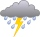A raindrop falls at a rate of 9.3 meters per second (m/s). Use the following facts to convert this speed to kilometers per hour (km/h). 1 km = 1000 m 1 min = 60 sec 1 hour = 60 min
• FrancescaFrancesca is growing 1/2 inch each year. How many years will it take for her to grow 6 inches?
• Water tank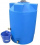The block-shaped tank is 2.5 m long, 100 cm wide and 12 dm high. In how many minutes will it be filled with water to two thirds if 40 liters of water flow into the tank per minute?
• ShortcutThe road from the cottage to the shop 6 km away leads either along a straight road that the bicycle can drive at a speed of 18 km/h or by "shortcut". It measures only 3.6 km. But the road from the cottage is all uphill - at a speed of 8 km/h, you can go h
• Cindy runSuppose it takes 1/3 of an hour for Cindy to run 3/5 of a mile. How many miles does she run in 1 hour?
• Brno-Tachov highwayThe distance Brno-Tachov is 360 km. At 8:00 a. M. , a car travels from Brno on the highway, traveling at an average speed of 90 km/h. An hour later, a car leaves Tachov towards Brno, whose average speed is 110 km/h. Calculate how far apart they will be at
• Škoda carsAt 8:30 am, Škoda 120 started from Prievidza at 60 km/h in the direction of Bratislava. At 9:00 started from Prievidza at the same direction Škoda Rapid at 90 km/h. Where and when will Škoda Rapid catch up Skoda 120?
• The caravans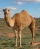At 6:00 in the morning, a caravan set out of the oasis at a speed of 1.5 miles per hour. Two hours later, a second caravan followed her at 2.5 miles per hour. When did the faster caravan run slower? How far from the oasis?
• Earth rotation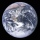How fast is the place on the Earth's equator moving if the Earth's radius is 6378 km?
• Athletic oval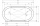On the 400 m athletic oval, two athletes started back to back from the same level. After the starting shot, they both ran away from each other at the same time. Athlete A ran at 15 km/h, and Athlete B ran at 17 km/h. A) in how many seconds did the athlete
• A bus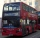A bus is scheduled to travel a distance 121 km in 2 3/4 hours. What average rate of speed must be maintained to arrive on schedule?
• An airplaneAn airplane flies 1440 km in 2 1/4 hours. What is its average speed in km per hour?
• On a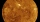On a some day, the Sun, Venus and the Earth are in eclipse, i. E. Venus is between the Sun and the Earth. Venus orbits the Sun in 225 days. In how many years will all three bodies be in alignment again?
• Cable carThe cable car will carry 1280 passengers in 40 minutes. How many people will be transported in 2.5 hours?
• The convoy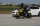During a military exercise, the convoy left the camp at 5 hours at a speed of 40 km/h. Half an hour later, a motorcycle clutch was sent at a speed of 70 km/h. How long before the motorcycle clutch reaches the column?
• Magnitude of angleWhat magnitude has an obtuse angle enclosed by the hands of clocks at 12:20 hours?

Do you have an exciting math question or word problem that you can't solve? Ask a question or post a math problem, and we can try to solve it.

We will send a solution to your e-mail address. Solved examples are also published here. Please enter the e-mail correctly and check whether you don't have a full mailbox.

Do you want to convert velocity (speed) units? Velocity - math problems. Examples for 7th grade (seventh).